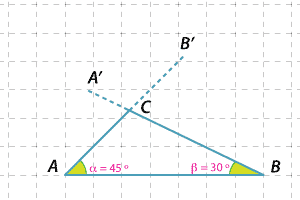### The AAS congruence test

• If two angles and one side of a triangle are respectively equal to two angles and the matching side of another triangle, then the two triangles are congruent.
• If we are given the angle sizes of a triangle and the length of a specific side, then only one such triangle can be constructed up to congruence.

The initials AAS stand for 'Angle', 'Angle', 'Side'.
In the construction below:
$$AB$$ is 7 units in length.
$$\angle B' AB = 45 ^\circ \text{and} \ \angle A' BA = 30 ^\circ$$ are drawn.
The segments AB′ and BA′ intersect at C.Detailed description

#### Question 5

Triangle $$XYZ$$ is drawn with $$XZ \ = 6 \text{cm and} \ \angle YXZ = 46 ^\circ \text{and} \ \angle YZX = 55 ^\circ$$.

#### Question 6

Construct a triangle $$ABC \ \text{with} \ AB = 5 \text{cm and} \ \angle CAB = 50 ^\circ \text{and} \ \angle CBA = 45 ^\circ$$.